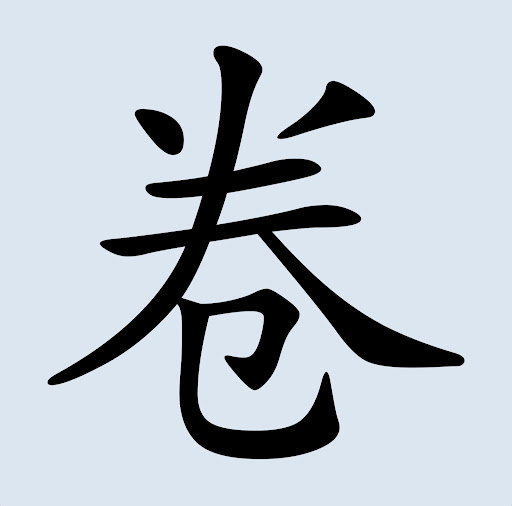komodovaran_ - 2 years ago 138
R Question

# Coloring facets of histograms in ggplot by grouping variable

I thought this would be an easy one, but not quite so.

I have my code for some histograms in facets. They can follow the standard color theme no problem, but if I want to have them follow a palette (which works fine for e.g. a single scatterplot with dots from different groups) everything goes haywire.

And where the heck does the red come in?

Data here: https://pastebin.com/0p7SP005

``````library(ggplot2)
library(ggthemes)

ggplot(data = point_list, aes(x = lifetime,
y = ..density..)) +
geom_histogram() +
aes(fill = as.factor(cluster),
col = "black") +
scale_x_continuous(expand = c(0,0)) +
scale_y_continuous(expand = c(0,0)) +
coord_cartesian(xlim = c(-2.6,50),
ylim = c(0,0.16)) +
theme_tufte(base_size = 11, base_family = "Helvetica") +
theme(axis.text = element_text(color = "black"),
panel.border = element_rect(colour = "black", fill=NA, size=0.7),
legend.position = "none") +
facet_wrap(~cluster, scales = "free", ) +
scale_color_brewer(palette = "Set1")
``````For reference, Set1 should look like this:Z.Lin

I'll illustrate the point about why the bars' outlines were red, since everything else has been covered already.

In your code, you had `col = "black"` inside the aesthetic mapping function, which meant that color (used for outlines) is mapped to a variable. "black" in this case would be interpreted as a factor with 1 level. Since your code also includes `scale_color_brewer(palette = "Set1")`, the resulting color is the first color from the Set1 palette, i.e. bright red.

(The actual word didn't matter; if you had `col = "white"` or anything like that, it would make no difference at all.)

If you had chosen a different palette, the outline color would be different too. For example:

``````ggplot(data = point_list, aes(x = lifetime,
y = ..density..)) +
geom_histogram(size = 3) + # thicker outline to make the color more obvious
aes(fill = as.factor(cluster),
col = "black") +
scale_x_continuous(expand = c(0,0)) +
scale_y_continuous(expand = c(0,0)) +
coord_cartesian(xlim = c(-2.6,50),
ylim = c(0,0.16)) +
# theme_tufte(base_size = 11, base_family = "Helvetica") + #commenting this out since I don't have this theme
theme(axis.text = element_text(color = "black"),
panel.border = element_rect(colour = "black", fill=NA, size=0.7),
legend.position = "none") +
facet_wrap(~cluster, scales = "free", ) +
scale_color_brewer(palette = "Set2")
``````The first color from the Set2 palette is pale-ish green (#66c2a5), which is what we see here.

To get black outlines, follow loki's answer above to specify the color in `geom_histogram`, and make sure you do it outside `aes()` this time. :)

Recommended from our users: Dynamic Network Monitoring from WhatsUp Gold from IPSwitch. Free Download